﻿ 基于时域解析信号的广义MUSIC算法
 舰船科学技术2017, Vol. 39Issue (10): 107-111PDF

Generalized MUSIC algorithm based on time-domain analytical signal
LI Zhi-zhong, XU Zhong-liang, CHEN Zhe, CHENG Yu-sheng
Navy Submarine Academy, Qingdao 266100, China
Abstract: A new generalized MUSIC beam forming algorithm based on time-domain analytical wideband signal was proposed. The algorithm combined time-domain analytical signal with the improved MUSIC algorithm, which generated a complex time analytical signal by Hilbert transformer after accurate time delay from wideband signal, and increased the weight vector degrees of freedom. A new generalized noise subspace was established by adding the adjusting index, which removed influences of conventional algorithm and improved the robustness. Finally, the DOA was estimated by orthogonal character of signal subspace and noise subspace. The results of simulation data and sea trial data show that the algorithm can get sharper peaks, improve the target angular resolution and improve the ability of weak target detection.
Key words: array signal processing     time-domain analytical signal     MUSIC
0 引　言

1 时域解析信号MUSIC算法原理 1.1 常规算法

 ${x_m}(t) = \sum\limits_{d = 1}^D {{s_d}(t - {\xi _m}({\theta _d}))} + {v_m}(t)\text{，}$ (1)

 ${\hat{ R}_f}({f_j}) = \frac{1}{K}\sum\limits_{k = 1}^K {{{X}_k}(j){X}_k^H} (j),\;\;(j = 1, \cdots J)\text{，}$ (2)

1.2 时域解析信号协方差矩阵

 ${Y} = {{Y}_s} + {{Y}_v} = {X} + j \cdot \bar{ X}\text{，}$ (3)

M个阵元的阵列接收信号经A/D采样、数字时延 $\Delta {\tau _i}(1 \leqslant i \leqslant M)$ 以及分数时延滤波之后的期望信号构造时域复数信号为：

 $Y = X + jH(X)\text{，}$ (4)

 $\hat R = \frac{1}{N}\sum\limits_{i = 0}^{N - 1} {Y{Y^H}}\text{，}$ (5)

 $C = {{W}^H}{Y} = {{W}^H}{{Y}_s} + {{W}^H}{{Y}_v}\text{，}$ (6)

 $P = E\{ C{C^*}\} = {{W}^H}{RW}{\text{。}}$ (7)
1.3 基于时域解析信号的广义MUSIC算法

 $P(\theta ) = \frac{1}{{{B^H}U{U^H}B}}\text{，}$ (8)

 $B = {\left[ {1,1,\cdots,1} \right]^{\rm T}}\text{，}$ (9)

 $G = \left[ \displaystyle{\frac{{{\nu _{D + 1}}}}{{\lambda _{D + 1}^p}},\frac{{{\nu _{D + 2}}}}{{\lambda _{D + 2}^p}},\cdots,\frac{{{\nu _M}}}{{\lambda _M^p}}} \right]\text{。}$ (10)

 ${P_G}(\theta ) = \frac{1}{{{B^H}G{G^H}B}}{\text{。}}$ (11)

2 仿真数据分析

2.1 仿真1单目标仿真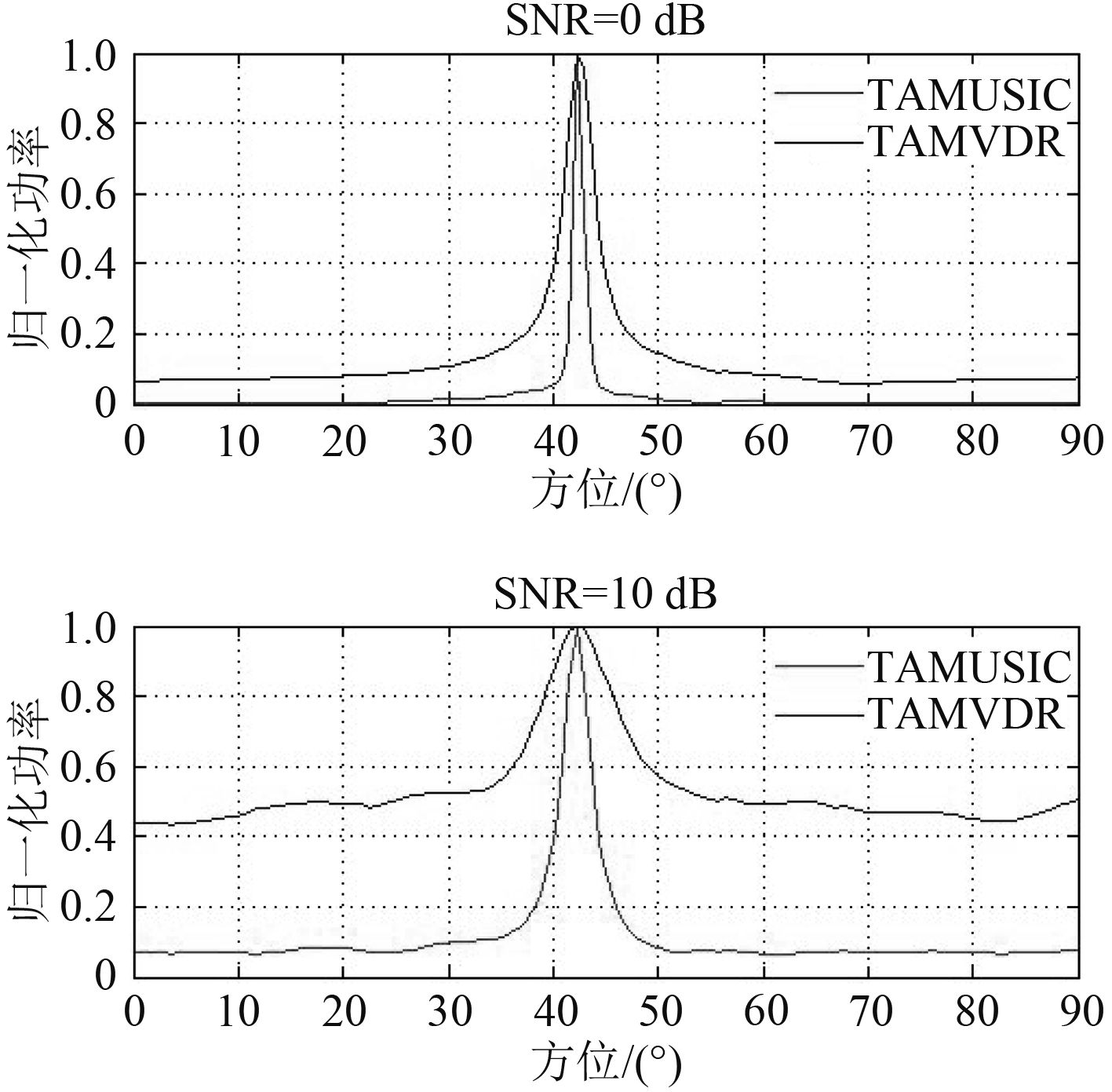图 1 不同信噪比时TAMVDR与TAMUSIC对比 Fig. 1 The comparison with different SNR
2.2 两目标仿真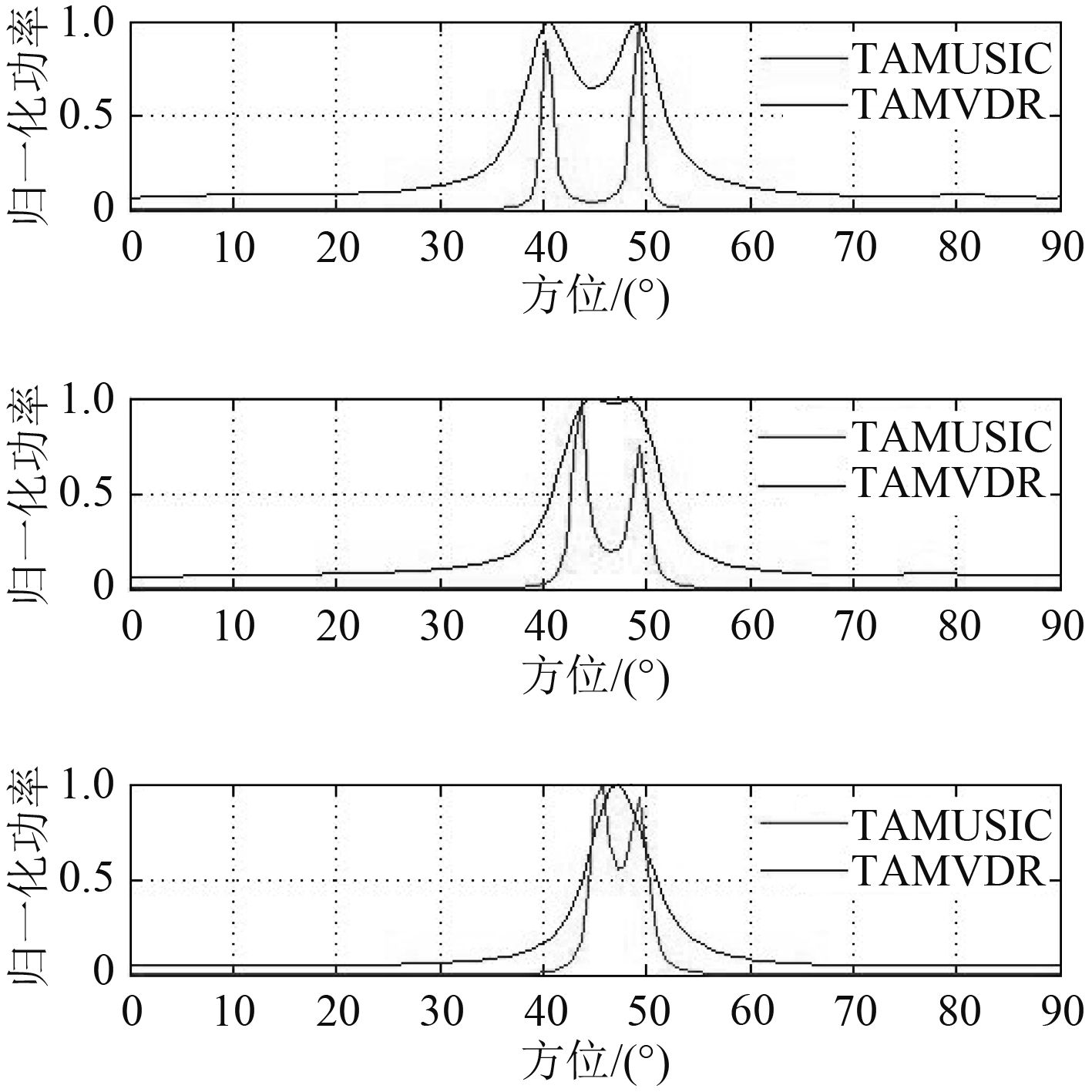图 2 不同角度间隔时TAMVDR与TAMUSIC对比 Fig. 2 The comparison with different space

3 海试数据分析

1）单快拍数据对比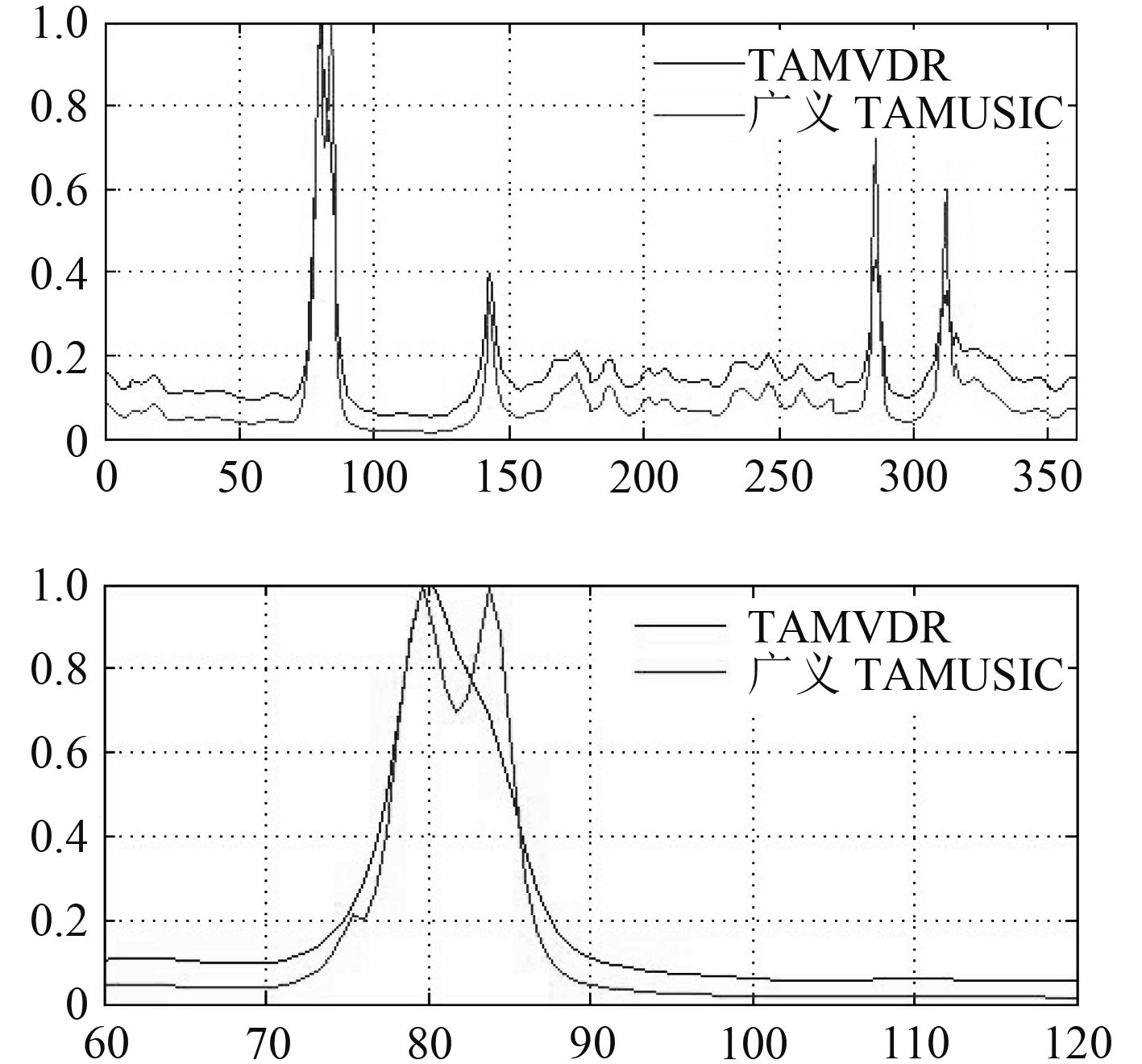图 3 第1 380次快拍输出 Fig. 3 The 1 380 th output

2）历程数据对比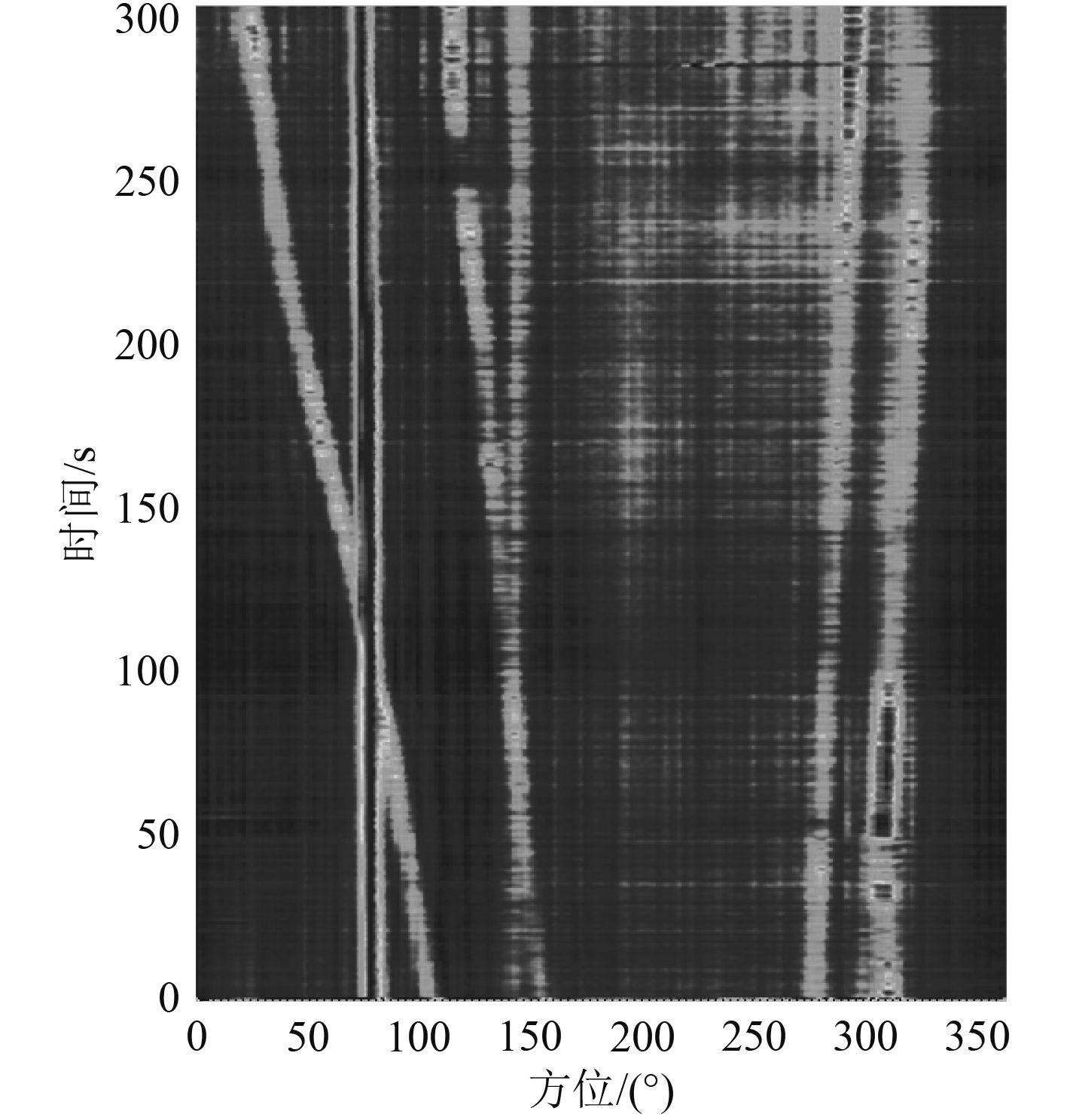图 4 CBF时间-历程图 Fig. 4 The CBF time-course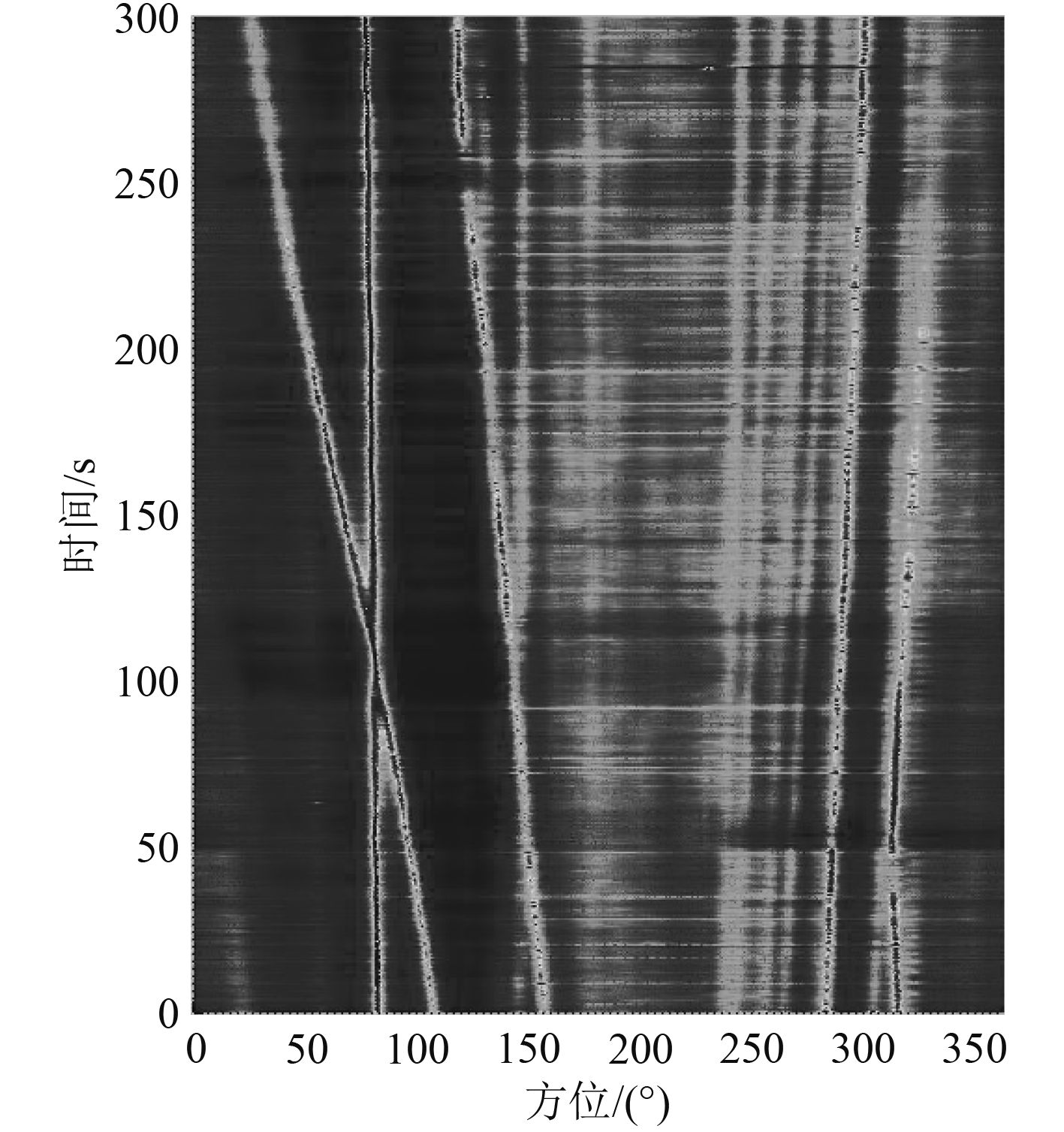图 5 TAMVDR时间-历程图 Fig. 5 The TAMVDR time-course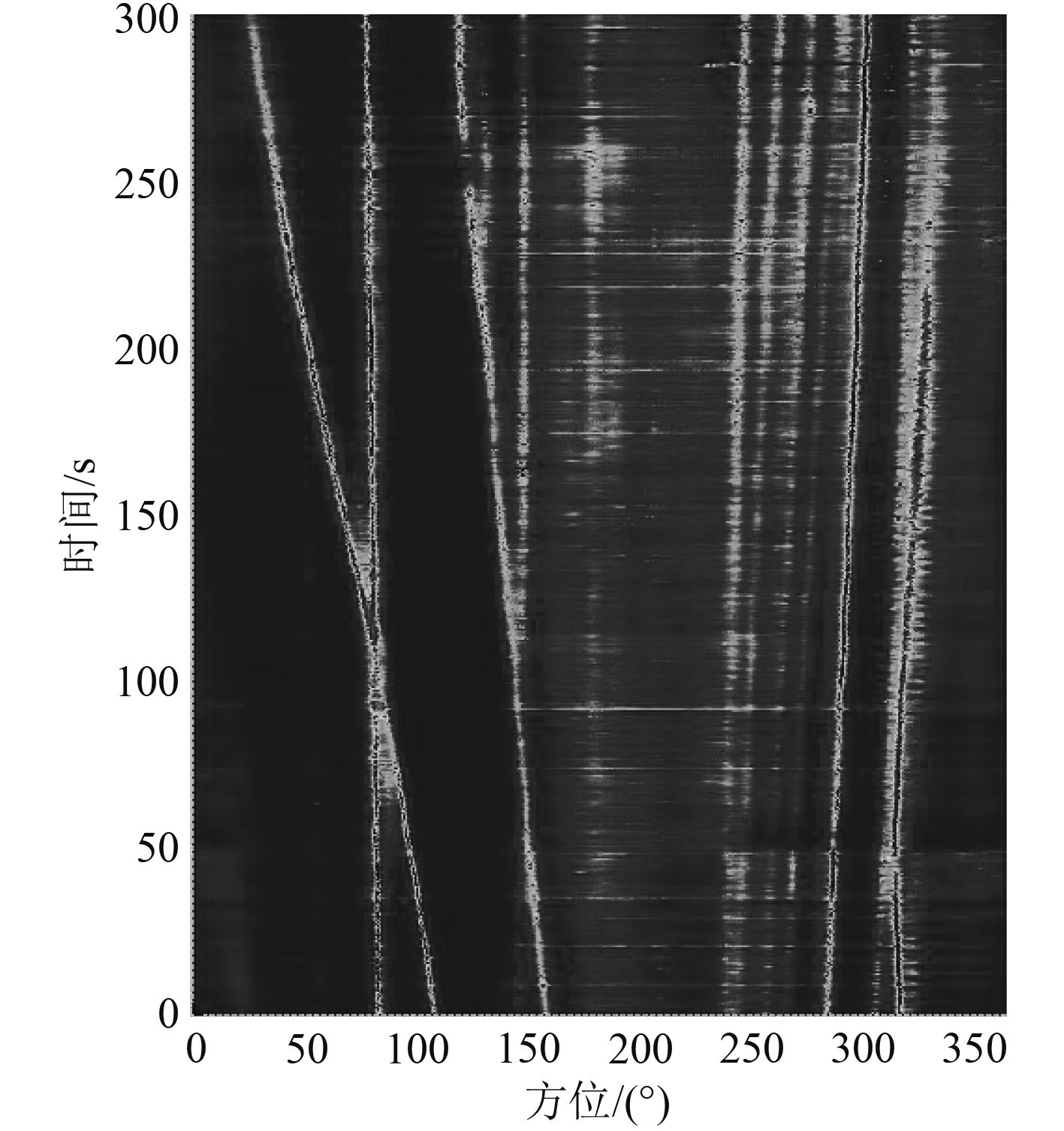图 6 TAMUSIC时间-历程图 Fig. 6 The TAMUSIC time-course

4 结　语

1）TAMUSIC算法继承了TAMVDR算法时域解析信号的复数加权优势，增加了权向量自由度；获得良好的波束形成处理效果。

2）TAMUSIC算法无需对多个快拍进行频域子带分解，只需对一次快拍进行处理即可求得最优解，大大减少了运算量。

3）TAMUSIC算法继承了传统MUSIC算法分辨率高的优点，实现了优于TAMVDR的目标分辨和检测能力。

4）TAMUSIC算法通过构建新的广义噪声子空间，克服了信源数目估计不准确对结果的影响，提升了在信源数目未确知条件下的目标探测能力，并改变调节指数的权重能改变弱目标相对强度，提高对小目标的发现能力。

  PALANISAMY P, KALYANASUNDARAM N. A new DOA estimation algorithm for wideband signals in the presence of unknown spatially correlated noise[J]. Signal Processing, 2009, 89 (10): 1921–1931. DOI: 10.1016/j.sigpro.2009.03.033  BYUN-CHUL K, ITAI L. High resolution broadband beam forming based on the MVDR method [C] // OCEANS 2000 MTS/IEEE Conference and Exhibition , 2000, 3(9): 1673–1676.  LO K W. Adaptive array processing for wide-band active sonar[J]. IEEE Journal of Oceanic Engineering, 2004, 29 (3): 837–846. DOI: 10.1109/JOE.2004.833096  SCHMIDT R O. Mutiple emitter location and signal parameter estimation[J]. IEEE Trans. AP, 1986, 34 (3): 276–280. DOI: 10.1109/TAP.1986.1143830  WONG K T, ZOLTOWSKI M D. " Self-lnitiating MUSIC-based direction finding in underwater acoustic Particle velocity-field beamspace”, IEEE Intl[J]. Symposium on Circuits & Systems, 1997 (4): 2553–2556.  STOICA P. Extended derivations of MUSIC in the presence of steering vector errors[J]. IEEE Trans. Signal Processing, 2005, 53 (2): 1209–1211.  WONG K T, ZOLTOWSKI M D. Root MUSIC based azimuth elevation angle of arrival estimation with uniformly space but arbitrarily oriented velocity hydrophones[J]. IEEE Trans Signal Processing, 1999, 47 (12): 3250–3260. DOI: 10.1109/78.806070  FEIFEI G, NALLANATHAN A, YIDE W. Improved MUSIC under the coexistence of both circular and noncircular sources[C]// Signal Processing, IEEE Transactions on. 2008, 56(7): 3033–3038.  刘伟, 王昌明, 张自嘉. 水声定位系统中空间谱估计算法仿真分析[J]. 电子测量技术, 2008, 31 (11): 47–49. LIU W, WANG C M, ZHANG Z J. Simulation analysis of spatial spectrum for underwater target azimuth estimation[J]. Electronic Measurement Technology, 2008, 31 (11): 47–49. DOI: 10.3969/j.issn.1002-7300.2008.11.014  WANG. Coherent signal-subspace processing for the detection and estimation of angles of arrival of multiple wideband sources[J]. IEEE. Trans. on Acoustics, Speech and Signal Processing, 1985, 33 (4): 823–831. DOI: 10.1109/TASSP.1985.1164667  冯西安. 水下目标高分辨方位估计技术研究[D]. 西安: 西北工业大学, 2004. FENG Xi-an. Study on the high resolution DOA estimation techniques of underwater targets[D]. Xi’an Northwestern Polytechnical University, 2004.  王良, 宋志杰, 华洋. 时域解析信号的MVDR自适应波束形成方法[J]. 数据采集与处理, 2009, 24 (3): 318–322. WANG L, SONG Z J, HUA Y. Adaptive MVDR beam forming algorithm based on time-domain analysis signals[J]. Journal of Data Acquisition & Processing, 2009, 24 (3): 318–322.  王良. 时域解析信号的自适应波束形成方法研究[D]. 青岛: 中国海洋大学, 2009. WANG Liang. Study on adaptive beam forming algorithm based on time-domain analysis signals[D]. Qingdao: Qingdao Ocean University of China, 2009.  许忠良, 杨威武, 李智忠. 基于分数时延的TAMVDR宽带波束形成方法[J]. 信号处理, 2012, 28 (12): 52–55. XU Z L, YANG W W, LU zz. TAMVDR wide-band beam-forming method based on fractional delay[J]. Signal Processing, 2012, 28 (12): 52–55.  张家平, 刘青, 张洪顺. 低信噪比中MUSIC算法研究[J]. 通信技术, 2009, 42 (1): 87–89. ZHANG J P, LIU Q, ZHANG H S. MUSIC algorithm with small SNR[J]. Communications Technology, 2009, 42 (1): 87–89.  李智忠, 许忠良, 李海涛, 等. 基于傅里叶变换的快速TAMVDR算法[J]. 舰船科学技术, 2016, 38 (1): 85–89. LI Z Z, XU Z L, LI H L, et al. Fast TAMVDR algorithm based on fourier transform[J]. Ship Science and Technology, 2016, 38 (1): 85–89.